# Binary Search

Try out this game before beginning:

Well, you must have thought of some strategy to find the number in an optimal way. Iterating through all the numbers is not possible within the given constraints.

Let’s look at one of the Strategies ( I guess the only one that is intuitive )

At its most fundamental level, a Binary search is employed to find a value in a sorted sequence.

The Pseudo-Code for a Binary Search Algorithm, where A is a sorted array.

`Binary_Search(A, value):      beg=1 , end = A.size      while ( beg <= end ):             mid = floor((beg+end)/2 )             if ( A[mid] == value ):                 Return mid; // Return the position of the value             else if ( A[mid] < value ):                 beg = mid+1;  // Search the upper half of the array             else:                 end = mid-1;  // Search the lower half of the array      return -1;      // Indicates target not found`

If you analyze the pseudo code properly, you will realize that at each execution of the loop the array to search becomes half, making the time complexity O(logN). This means that even if the array is of the size 10⁷, you can find the value in 25 executions.

## Let’s take the following example

We have to find the value -23.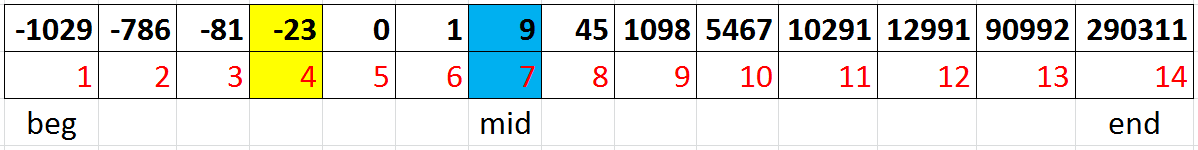Initially we start off with beg = 1 , end = 14 and mid = (1+14)/2 = 7

Here since Arr[mid] > Search_Value ; we discard the right side of the array.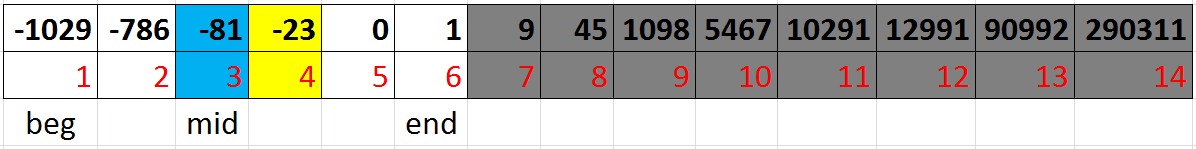After discarding the right (greater than equal to mid) side of the array, beg = 1, end = 6 and mid = ( 1 + 6 ) / 2 = 3

Here since Arr[mid] < Search_Value ; we discard the left side of the array.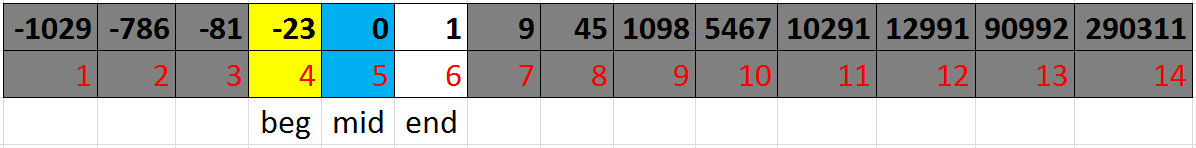After discarding the left (lesser than equal to mid) side of the array, beg = 4, end = 6 and mid = ( 4 + 6 ) / 2 = 5

Here since Arr[mid] > Search_Value ; we discard the right side of the array.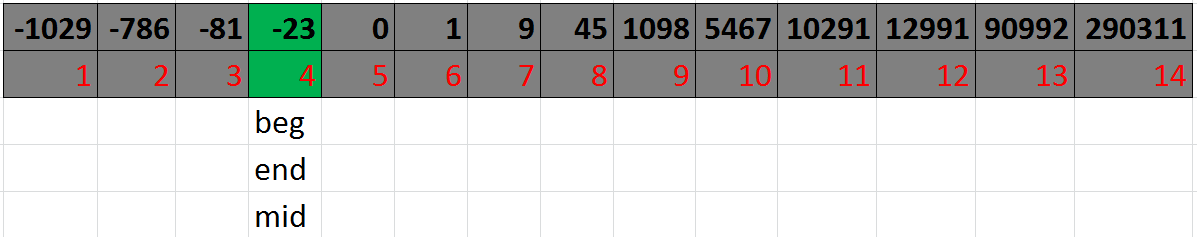After discarding the right (greater than equal to mid) side of the array, beg = 4, end = 4 and mid = ( 4+ 4 ) / 2 = 4

Here, since Arr[mid] == Search Value ; Bingo, we have found the value !!

Notice how the range becomes shorter and shorter after each execution! This is what makes the binary search algorithm so powerful.

If we had to search for an element that is not there, the range would become shorter and shorter till only one element remains, after which it comes out of the loop and returns -1.

## STL Functions for binary search

STL offers functions to implement binary search in an efficient way. Try exploring the functions binary_search(), lower_bound(), upper_bound().

## Expanding Further

Now, a binary search can do way more things than just find a value in an array of integers. It can be used wherever there is a monotonous function that has either a true or a false value.

Let’s suppose f(x) is a boolean function which returns either true or false. In a problem, you encounter such a function.
Now you want to test whether a binary search is applicable or not. What is the required condition?

In simple terms, a binary search can be used whenever a boolean predicate function (a yes or no function) is monotonous (i.e) has a series of yes followed by a series of no or the vice-versa.
In this case, binary search can be used to determine where the transition takes place. This comes quite handy.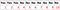The output of a predicate function f(x) on the indices of an array

Now, you want to determine the max value of x for which f(x) gives Yes.
You can employ the following pseudo-code.

`Abstract_binary_search( lower_l , upper_l )          beg = lower_l , end = upper_l        while (beg <= end):              mid = floor((beg+end)/2 )              if( f(mid) = true ): // f(x) is the predicate function                 beg = mid+1              else:                 end = mid-1        return end  // returns the last value for which f(x) is Yes`

Let’s analyze what is happening.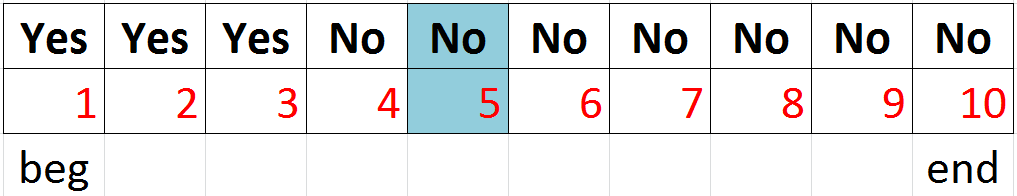Initially we start off with beg = 1 , end = 10 and mid = (1+10)/2 = 5

Here since f(mid) = No, we discard the right of the array ( including the mid) and move leftwards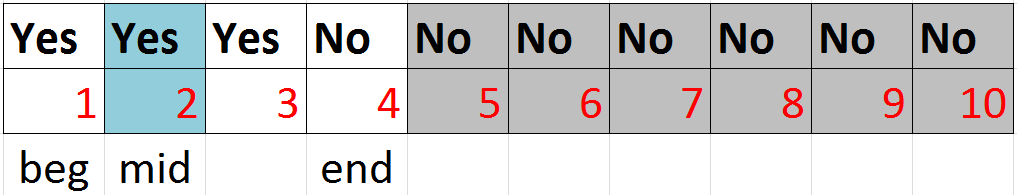After discarding the right (No) side of the array, beg = 1, end = 4 and mid = ( 1 + 4 ) / 2 = 2

Here since f(mid) = Yes, we discard the left of the array ( except the rightmost element on the left ) and move rightwards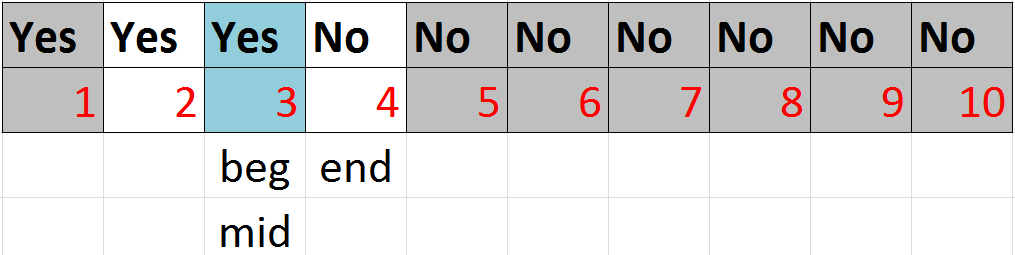After discarding the left (Yes) side of the array, beg = 3, end = 4 and mid = ( 3 + 4 ) / 2 = 3

Here since f(mid) = Yes, we discard the left of the array ( except the rightmost element on the left ) and move rightwards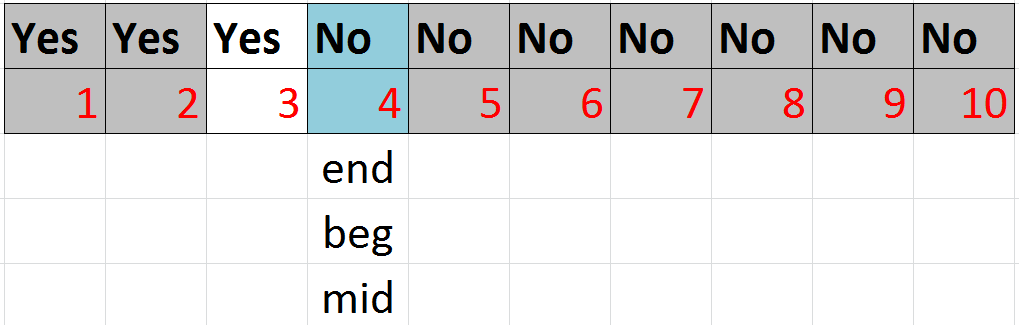After discarding the left (Yes) side of the array, beg = 4, end = 4 and mid = ( 4 + 4 ) / 2 = 4

Here since f(mid) = No, we discard the right of the array ( including the mid) and move leftwards

Now since beg > end it breaks out of the loop.
Also beg points to the first No, and end points to the first Yes.

As you can see, since this is a function, this binary search doesn’t require an array or sequence of integers and hence is not limited by memory space. Even a billion integers can be explored in 30 steps, because of its O(logN) complexity.

This can be applied to a wide range of problems. Let’s take the example of Aggressive Cows.

The necessary thing to solve this question is proper observation & then working on how to choose the predicate function so that we can apply binary search. Suppose X is the maximum possible separation between the cows.

Then ∀ j < X, it is possible to arrange the cows with a separation of j and
∀ j > X, it is not possible to arrange the cows with a separation of j.
This means that we can try to choose a monotonous function which might help us to solve the question with a binary search approach.

Now, if we choose F(x) such that it gives:
True, if it is possible to arrange the cows with at least X distance between, and False otherwise.
So we have a series of Yes followed by No, and we need to find the maximum value of the occurrence of Yes. Doesn’t this sound familiar?

Let’s take a look at the pseudo code.

`f(A, dist, cows):     n = A.size     i = 1      // Holds stall in which last cow is put                // Here cow1 is put in stall1           for ( j in (2...cows) ) :         k = i+1         while ( ( k<=n ) and ( A[k]-A[i] < dist ) ):                 k = k+1  // finding the stall with distance >= dist         if (k == n+1):                 Return false // if not found, this dist is not poss         i = k    // if found, put next cow in it i.e. j in it          j = j+1  // move on to next cow      if (j > cows): // if all the cows have been put, dist is poss         return true     else:         return falseSolve( A, cows ):     n = A.size     sort(A)     beg = 1, end = ( A[n]-A )     While ( beg<=end ):           mid = floor((beg+end)/2 )           if ( f(A, mid, cows) == true ):                   beg = mid+1           else :                   end = mid-1     return end`

The analysis of the pseudo code and its time complexity is left as an exercise for the reader.

https://www.codechef.com/problems/SNAKEEAT

https://www.codechef.com/problems/STACKS

https://www.spoj.com/problems/SUBS/

https://www.codechef.com/problems/FORESTGA

https://www.interviewbit.com/courses/programming/topics/binary-search/

https://www.codechef.com/IOITC181/problems/CIRCINTE

You should definitely go through this for some more detailed insights:

Written by Kousshik Raj, Himanshu Mundhra